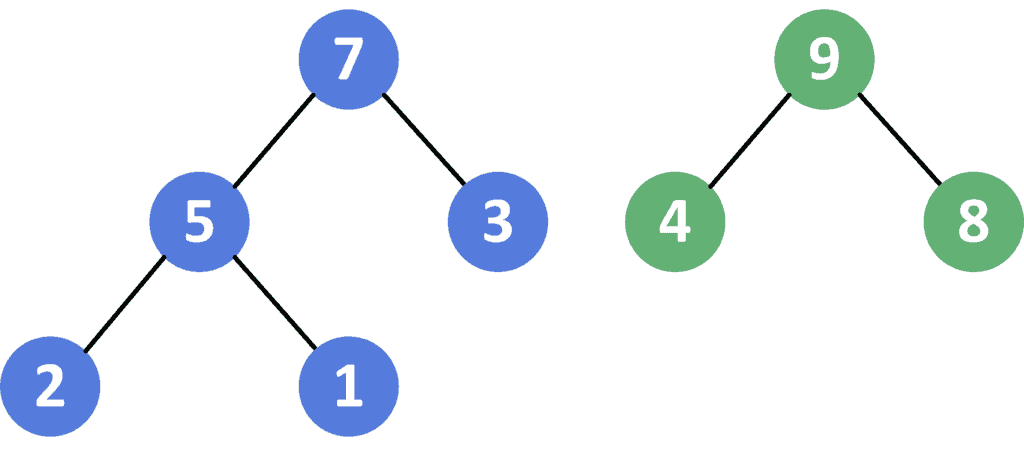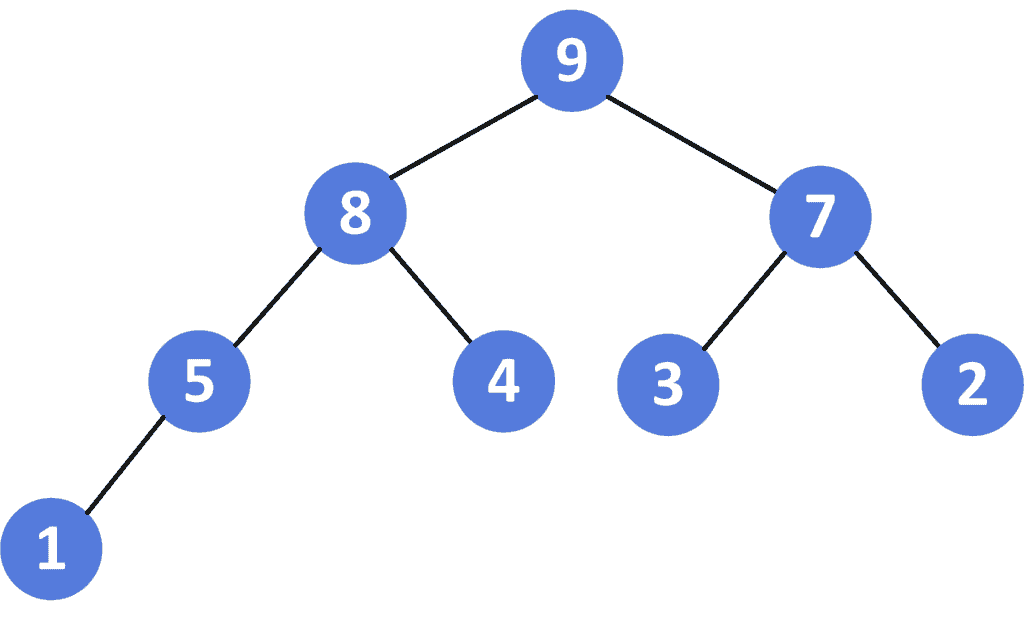## 1. Overview

In this tutorial, we’ll talk about the problem of merging two max binary heaps.

First, we’ll define the problem and provide an example to explain it. Then, we’ll present a solution for this problem and work through its implementation and time complexity.

## 2. Defining the Problem

Suppose we have two max binary heapsand, and we want to merge them into a single max heap. Recall the max binary heap is a binary tree where each node in this tree has a value greater than all the nodes’ values in its subtree.

Let’s take a look at the following example for a better understanding, Given two max binary heapsandin array representation. Recall we can represent a binary tree in an array format where the node with indexhas its left and right children located onandrespectively:

•:•:###After merging these two max binary heaps, we’ll get the following one:

•## 3. Solution

### 3.1. Main Idea

The main idea is to merge the array representation of the given max binary heaps; then we build the new max heap from the merged array.

First, we fix one of the given max heaps as a solution. Then, we’ll append the elements of the other max heap to it. Second, we’ll build a max heap on the merged array.

Finally, the max heap will be the merged version of the two given max heaps.

### 3.2. Max Heapify

Let’s take a look at the implementation of the algorithm:Initially, we have the recursive functionthat will rearrange the elements of a given array to make the heap valid. The function will have two parameters: the array of the heap we want to buildand the current position.

First, we declareandchildren of the current node, which will be equal toandrespectively. Then, we’ll declare, which represents the index of the node that has the maximum value.

Second, we check if there’s anode. If there’s one and its value exceeds the current max node, we update theto equal. Also, we do the same for thechild.

Finally, we check ifisn’t equal to the current, which means there’s a child ofnode with a value greater than its value. If so, we swap them to make the heap valid and call our function to the newto check if it’s still valid.

### 3.3. Algorithm

Let’s take a look at the implementation of the algorithm:Initially, we have thefunction that will return the merged max heap from the given two max heaps. The function will have two parameters, the first and the second max heaps.

First, we declarethat will store the array representation of the merged version of the given max heap. Initially, it’s equal to the first max heap.

Second, we iterate over the elements of the second max heap’s arrayand append each element to the.

Third, we iterate over the nodes of the current heap in bottom-up order and callto fix the invalid nodes that don’t meet the definition of max heap.

Finally, we return the.

### 3.4. Complexity

The time complexity of this approach is, whereis the number of vertices of the first heap, andis the number of vertices of the second heap. The reason behind this complexity is the same as the complexity of building a max heap.

The space complexity here is. since we’re storing the vertices of the new heap.

## 4. Conclusion

In this article, we discussed the problem of merging two max binary heaps. We defined the problem and provided an example to explain it.

Finally, we provided a simple approach to solve it and walked through its implementation and time complexity.